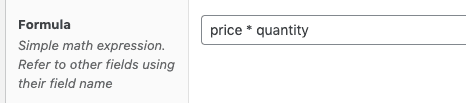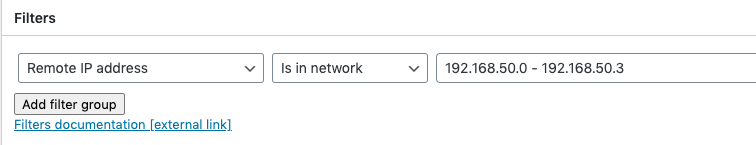# Expressions

Expressions are the corner stone of Calculated fields for ACF. It’s what you enter in to the “Formula” field that this plugin adds to the ACF field editor.The expressions syntax is designed to be as familiar to “normal usage” as possible, most people that have used Excel should be pretty comfortable with the way of entering expressions. An expression will always return a value when it is evaluated, if the expression contains errors, it will silently return 0 (zero).

You can think of the content of the formula field as an assignment expression, the field will get the return value of the expression in the formula field. If an expression can’t be evaluated due to invalid syntax or referring to undefined field names, it will silently return 0 (zero).

The valid building blocks of an expression are the following parts:

### Constants

This could be any number “hard coded” into the formula field. The simplest possible formula using a constant would be:This formula will of course always return the value 10, perhaps not very exciting.

### Built in constants

Calculated Fields for ACF also has built in named constants that can be used:The following built in constants are available:

Constant Description
pi The mathematical constant pi. 3.1415…..
e The mathematical constant e, 2.71828 is the base of the natural logarithm

Note that when using these constants, they will override any field names. If you name another field pi or any of the above built in constants, you will not be able to refer to that field in a formula.

### Fields (variables)

An expression can pick up a value from another field on the same post. This is done by simply referring to the other field via it’s ACF field name:The above expression will return the value of the field “price” to the calculated field. Note that when a field expression is evaluated the returned field value may also be the result of a previous calculation. Field expressions are calculated in the order that the fields appear in the ACF field editor. It’s therefore important that an expression only refers to fields that appear before / above it in the field order. If an expression refers to a field that is defined after / below it, the result will be unpredictable.

### Operators

Operators are the things that actually perform calculations. A very simple expression using an operator is:Part Description
price A field expression. This is the name of another field. When this part of the expression is evaluated, the string “price” will be replaced with the current.
* Multiplication operator. Multiplies the expression to the left with the expression to the right
10 A constant expression.

### Functions

Calculated Fields for ACF also supports simple functions as part of an expression. A function always has the form: name(parameter). An example expression containing a function:The above expression will return the value of price rounded to the nearest integer value. Note that all functions in Calculated fields for ACF always take exactly one (1) parameter, this is a simplification that might change in the future.

### Parentheses

For more detailed control of the calculation order, an expression can use parentheses. The content inside the parentheses is evaluated as a separate expression.

The expression`(2 + 10) * 3`is evaluated in two (2) steps. In step one (1), the expression in the parentheses is evaluated so it becomes: `12 * 3`

In step two (2), the resulting expression is evaluated`12 * 3 = 36`

Parentheses may be nested in as many levels as needed. For instance `((((12*4) * 2) *3) *4)` is a valid expression.